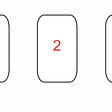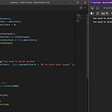# Understanding HyperLogLog

Imagine you go to a party and you want toknow how many people are at that party, how would you determine that? One way is to use brute force to manually and sequentially count each individual and get the total. But obviously, you don’t want to do that in a party, right? So, what are the other options? Just hold on for a moment and think about it.

Let’s assume you meet someone, and you ask his name, and the person replies “Tony”. You meet the next person and he introduces himself as “Steve”. At this point you think, what’s the probability that I meet a person whose name is “Tony” or “Steve”? It’s pretty high, since both these names are very common. So, how many people would you need to meet, to find someone whose name is “Tony” or “Steve”? It’s pretty low, right? For all you know, your next-door neighbor might have these names. But if you meet a person whose actual name is say “Iron Man” or “Captain America” (pardon my Marvel fandom), and following the same logic, you would get an interesting insight. To find someone, whose name is “Iron Man” or “Captain America”, you would probably need to talk to each person on earth and ask their names, since these names are just so rare (or borderline nonexistent). So, if you find someone with a name as rare as “Iron Man”, you are either meeting a person who had one too many, or you get a sense that the number of individuals in the party you are in, must be huge!

Okay, now putting the same understanding to binary numbers, assume you have a set of random binary numbers like:

`0011 0001 1001 1101 1111`

Here, let’s look at the number of preceding zeroes in each number:

`0011 - 2 0001 - 3 1001 - 0 1101 - 0 1111 - 0`

Now, let’s try to determine, to get a single preceding zero in a binary number, how many numbers would you need to scan through? The answer is 2. Because in a random distribution, half of the numbers would start form 0 and half would start from 1. Extending this further, the probability of getting 2 preceding zeroes would be 0.25, 3 preceding zeroes would be 0.125 and for k zeroes would be 1/(2^k). Consequently, to encounter a binary number with 2 preceding zeroes, you would need to scan through roughly 2², 3 would be 2³ and for k preceding zeroes, this count would be 2^k. This roughly forms the base of the HyperLogLog algorithm.

In the previous example, we could see that the maximum number of preceding zeroes was 3, which would mean there are roughly 2³ = 8 elements in our set. But there were actually 5. So, there’s a significant variance here. Obviously, if we have a larger and a randomized data-set, we would get a lower variance, but we still can’t trust this method as one freak entry would mess up the entire count (Similar to a situation where you are invited to a private party with Iron Man).

The solution to this would be to divide our set of numbers into various buckets. To decide these buckets, we could use any hash function. Let’s assume our hash function returns the last 2 digits of the input number. So, our buckets would look something like this:

`11 - 0011, 111101 - 0001, 1001, 1101 `

Now, we calculate the maximum number of preceding zeroes per bucket:

`11 - 2 01 - 3 `

Now, instead of simply taking the maximum i.e. 3, we take the mean of bucket-wise maximum number of preceding zeroes, which gives us a mean max of (2+3)/2 = 2.5, which brings our count to 2^(2.5) = 5.6, which is quite close to the actual number.

This algorithm can be used to calculate the cardinality of a set or the count of distinct elements in a set in a constant time and log(log(N)) space. It has been found experimentally that it gives a constant error percentage determined by

1.3/sqrt(m)

Where m is the number of buckets. In our case, since we had 3 buckets, our error rate would be 1.3/1.732 = 0.75 %, which is almost like saying that, if you have a million elements in your data-set, this algorithm would tell you that you have around 992500 to 1007500 elements, which should be very acceptable in many use cases.

To get more accurate results, we could simply ignore the top 30% buckets with the highest number of preceding zeroes, which has been found to bring the error rate down to

1.04/sqrt(m)

So, the next time you are at an event and are curious to know, how many people are you surrounded with, start with asking names!

Ref:

--

--

--

## More from Utkarsh Upendra

Software Developer @ Optum, interested in Design Patterns, Algorithms, Data Systems and Statistical Methods, currently exploring BPMN and DMN using Camunda.

Love podcasts or audiobooks? Learn on the go with our new app.

## Monty-Hall Problem(with python simulation)## Differential Equations 10: The Nonhomogeneous Equation & Constant Coefficient Homogeneous Equations## The Inclusion-Exclusion Principle & The Proof of Euler’s Phi Function## Is your number really random?## Spider’s Web Puzzle## Differential Equations 32: The Frobenius Method## Utkarsh Upendra

Software Developer @ Optum, interested in Design Patterns, Algorithms, Data Systems and Statistical Methods, currently exploring BPMN and DMN using Camunda.

## Single Responsibility Principle